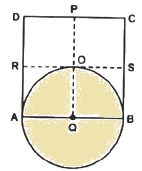Guru

# Prove that the circle drawn with any side of a rhombus as diameter, passes through the point of intersection of its diagonals. Q.5

• 0

What is the simplest way for solving the question of class 9th of exercise 10.6 of math of question no.5 give me the best and simple way for solving this question in simple way Prove that the circle drawn with any side of a rhombus as diameter, passes through the point of intersection of its diagonals.

Share

1.To prove: A circle drawn with Q as centre, will pass through A, B and O (i.e. QA = QB = QO)

Since all sides of a rhombus are equal,

AB = DC

Now, multiply (½) on both sides

(½)AB = (½)DC

So, AQ = DP

BQ = DP

Since Q is the midpoint of AB,

AQ= BQ

Similarly,

RA = SB

Again, as PQ is drawn parallel to AD,

RA = QO

Now, as AQ = BQ and RA = QO we get,

QA = QB = QO (hence proved).

• 0